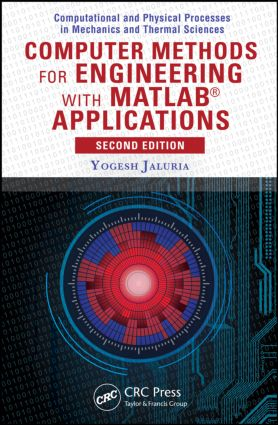# Computer Methods for Engineering with MATLAB® Applications

## 2nd Edition

CRC Press

632 pages | 179 B/W Illus.

Hardback: 9781591690368
pub: 2011-09-08
SAVE ~\$29.00
\$145.00
\$116.00
x
eBook (VitalSource) : 9780429185076
pub: 2011-09-08
from \$72.50

FREE Standard Shipping!

### Description

Substantially revised and updated, Computer Methods for Engineering with MATLAB® Applications, Second Edition presents equations to describe engineering processes and systems. It includes computer methods for solving these equations and discusses the nature and validity of the numerical results for a variety of engineering problems. This edition now uses MATLAB in its discussions of computer solution.

New to the Second Edition

• Recent advances in computational software and hardware
• A large number of MATLAB commands and programs for solving exercises and to encourage students to develop their own computer programs for specific problems
• Additional exercises and examples in all chapters
• New and updated references

The text follows a systematic approach for obtaining physically realistic, valid, and accurate results through numerical modeling. It employs examples from many engineering areas to explain the elements involved in the numerical solution and make the presentation relevant and interesting. It also incorporates a wealth of solved exercises to supplement the discussion and illustrate the ideas and methods presented. The book shows how a computational approach can provide physical insight and obtain inputs for the analysis and design of practical engineering systems.

Introduction

Introductory Remarks

Numerical Solutions

Importance of Analytical Results

Physical Considerations

Application of Computer Methods to Engineering Problems

Outline and Scope of the Book

Basic Considerations in Computer Methods

Introduction

Computational Procedure

Numerical Errors and Accuracy

Iterative Convergence

Numerical Parameters

A Review of MATLAB Programming

Introduction

MATLAB Environment

Ordinary Differential Equations

Input/Output

Script m-Files

Function m-Files

Plotting

Taylor Series and Numerical Differentiation

Introduction

The Taylor Series

Direct Approximation of Derivatives

Taylor-Series Approach and Accuracy

Polynomial Representation

Partial Derivatives

Roots of Equations

Introduction

Search Method for Real Roots

Bisection Method

Regula Falsi and Secant Methods

Newton–Raphson Method and Modified Newton’s Method

Successive Substitution Method

Other Methods

Numerical Solutions of Simultaneous Algebraic Equations

Introduction

Gaussian Elimination

Gauss–Jordan Elimination

Compact Methods

Numerical Solution of Linear Systems by Matrix Inversion

Iterative Methods

Homogeneous Linear Equations

Solution of Simultaneous Nonlinear Equations

Numerical Curve Fitting and Interpolation

Introduction

Exact Fit and Interpolation

Lagrange Interpolation

Newton’s Divided-Difference Interpolating Polynomial

Numerical Interpolation with Splines

Method of Least Squares for a Best Fit

Function of Two or More Independent Variables

Numerical Integration

Introduction

Rectangular and Trapezoidal Rules for Integration

Simpson’s Rules for Numerical Integration

Higher-Accuracy Methods

Integration with Segments of Unequal Width

Numerical Integration of Improper Integrals

Numerical Solution of Ordinary Differential Equations

Introduction

Euler’s Method

Improvements in Euler’s Method

Runge–Kutta Methods

Multistep Methods

Predictor–Corrector Methods

Boundary-Value Problems

Numerical Solution of Partial Differential Equations

Introduction

Parabolic PDEs

Elliptic PDEs

Hyperbolic PDEs

Appendix A: Some Common Commands in MATLAB

Appendix B: Computer Programs in MATLAB

Appendix C: Computer Programs in FORTRAN

References

Index

A Summary and Problems appear at the end of each chapter.

Yogesh Jaluria is a Board of Governors Professor in the Mechanical and Aerospace Engineering Department at Rutgers University. He has contributed to more than 450 technical articles and received numerous honors, including the Kern Award from AIChE, the Max Jakob Memorial Award from ASME and AIChE, and the Robert Henry Thurston Lecture Award, Freeman Scholar Award, Worcester Reed Warner Medal, and Heat Transfer Memorial Award, all from ASME.